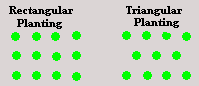Spike's Calculators

# Plant Spacing Multiplier Calculator (Metric)Find the multiplier for number of plants needed based on rectangular and triangular pattern planting.

This multiplier can be used to estimate the amount of plants needed for an area.

##### Example:
```Enter the amount of spacing required in the calculator.
Required plant spacing = 20 centimetres
The multiplier for 20cm plant spacing for rectangular pattern planting = 2.5
The multiplier for 20 cm plant spacing for triangular pattern planting =  2.89

An area of 10  metres by 3 metres for planting.
Square metres of area to be planted = 10m x 3m  =  30 sq metre

Multiply your square metres by the multiplier.
Estimated amount of plants needed for rectangular pattern planting 30 x 2.5 =  75 plants.
Estimated amount of plants needed for triangular pattern planting 30 x 2.89 =   87 plants.
```
Plant Spacing: cm

#### Results:

 Square Pattern Planting: # plants needed Triangular Pattern Planting: # of plants needed